# EpiInvertForecast

## Overview.

The COVID-19 epidemic has provided us with information on the evolution of the daily incidence in many different countries and epidemic scenarios. In this work we design a learning procedure, EpiInvertForecast, to forecast the future trend of the daily incidence from the evolution of other incidence curves that in the past were close to the current curve. We use a database with a collection of 27,418 restored incidence curves calculated by EpiInvert.

We forecast the restored incidence curve because the original incidence includes strong weekly administrative noise and is subject to strong disturbances. The restored incidence curve determines the incidence trend and it is much more stable and predictable than the original incidence. The usual way to provide stable and smooth incidence curves is to use the 7 or 14 days accumulated value of the daily incidence. As we show in the last section of this vignette, using the restored incidence curve provided by EpiInvert instead of the usual 7 or 14 days accumulated value of the daily incidence has a number of advantages.

Each of the 27,418 restored incidence curves in our database contains the values of the last 56 days of evolution obtained by EpiInvert using real data. We predict the evolution of the current restored curve in 2 optional ways : in the first option that we name by “mean” we make a weighted average of the 27,418 database curves where the weight of each curve depends on the similarity between the values of the first 28 days of the database curve and the last 28 days of the current curve. The last 28 days of the result of this weighted averaging give us the forecast of the current curve. In the second option, that we name “median”, we take the median of the closest curves, in the database, to the current incidence using the same similarity criterium.

We also compute empiric confidence intervals for the restored incidence forecast by applying the proposed method to our database and evaluating the forecast error accordingly with the number of days passed from the current day (the last day of the used incidence curve).

Finally, as EpiInvert also computes weekly administrative bias correction factors we obtain “for free”, a forecast of the original incidence curve by dividing the restored incidence curve by the corresponding correction factors. For a more detailed description of the method see EpiInvertForecast, 2022

## Package installation

EpiInvertForecast is included in the EpiInvert package. You can install the development version of EpiInvert from GitHub with:

 install.packages("devtools")
devtools::install_github("lalvarezmat/EpiInvert")

## Examples

We attach some required packages

library(ggplot2)
library(grid)
library(EpiInvert)
devtools::load_all(".")

Loading stored data on COVID-19 daily incidence up to 2022-05-05 for France, Germany, the USA and the UK:

data(incidence)
tail(incidence)
#>           date   FRA    DEU    USA    UK
#> 828 2022-04-30 49482  11718  23349     0
#> 829 2022-05-01 36726   4032  16153     0
#> 830 2022-05-02  8737 113522  81644    32
#> 831 2022-05-03 67017 106631  61743 35518
#> 832 2022-05-04 47925  96167 114308 16924
#> 833 2022-05-05 44225  85073  72158 12460

Loading some festive days for the same countries:

data(festives)
#>          USA        DEU        FRA         UK
#> 1 2020-01-01 2020-01-01 2020-01-01 2020-01-01
#> 2 2020-01-20 2020-04-10 2020-04-10 2020-04-10
#> 3 2020-02-17 2020-04-13 2020-04-13 2020-04-13
#> 4 2020-05-25 2020-05-01 2020-05-01 2020-05-08
#> 5 2020-06-21 2020-05-21 2020-05-08 2020-05-25
#> 6 2020-07-03 2020-06-01 2020-05-21 2020-06-21

Loading the restored incidence curve database used by EpiInvertForecast. This database contains the last 56 values of the restored incidence curves obtained by 27,418 executions of EpiInvert using real data. The format of this database is a 27,418 X 56 matrix. Each restored incidence curve in the database is normalized (multiplying by a scale factor) in order to the average of the first 28 values be equal to 1. To compare the curves of the database with the current curve we normalize the current curve in the same way.

data(restored_incidence_database)
#>          V1     V2     V3     V4     V5     V6     V7     V8     V9    V10
#> [1,] 1.8380 1.7586 1.6753 1.5853 1.4910 1.4000 1.3176 1.2450 1.1810 1.1240
#> [2,] 1.8150 1.7475 1.6766 1.6015 1.5190 1.4310 1.3460 1.2674 1.1980 1.1360
#> [3,] 1.7420 1.6970 1.6474 1.5940 1.5370 1.4740 1.4020 1.3230 1.2447 1.1717
#> [4,] 1.7194 1.6796 1.6336 1.5826 1.5277 1.4696 1.4070 1.3366 1.2610 1.1850
#> [5,] 1.6770 1.6450 1.6070 1.5630 1.5140 1.4610 1.4053 1.3450 1.2770 1.2040
#> [6,] 1.6260 1.6004 1.5703 1.5346 1.4930 1.4470 1.3970 1.3440 1.2860 1.2210
#>         V11    V12    V13    V14    V15    V16    V17    V18   V19    V20
#> [1,] 1.0720 1.0224 0.9744 0.9275 0.8820 0.8390 0.7980 0.7600 0.725 0.6930
#> [2,] 1.0800 1.0280 0.9785 0.9300 0.8830 0.8380 0.7950 0.7546 0.717 0.6830
#> [3,] 1.1054 1.0450 0.9885 0.9350 0.8840 0.8356 0.7896 0.7466 0.707 0.6704
#> [4,] 1.1145 1.0500 0.9915 0.9377 0.8875 0.8400 0.7950 0.7516 0.711 0.6740
#> [5,] 1.1310 1.0630 1.0015 0.9455 0.8943 0.8470 0.8020 0.7595 0.719 0.6810
#> [6,] 1.1510 1.0820 1.0170 0.9587 0.9060 0.8575 0.8130 0.7710 0.731 0.6930
#>         V21    V22    V23    V24    V25    V26    V27    V28    V29    V30
#> [1,] 0.6640 0.6375 0.6136 0.5920 0.5720 0.5540 0.5370 0.5203 0.5040 0.4876
#> [2,] 0.6520 0.6240 0.5990 0.5765 0.5564 0.5383 0.5220 0.5060 0.4910 0.4766
#> [3,] 0.6374 0.6080 0.5824 0.5604 0.5414 0.5250 0.5096 0.4957 0.4830 0.4704
#> [4,] 0.6400 0.6100 0.5830 0.5590 0.5385 0.5200 0.5040 0.4900 0.4770 0.4650
#> [5,] 0.6464 0.6150 0.5866 0.5614 0.5390 0.5197 0.5025 0.4870 0.4735 0.4610
#> [6,] 0.6574 0.6246 0.5947 0.5680 0.5440 0.5224 0.5035 0.4866 0.4715 0.4580
#>         V31    V32    V33    V34    V35    V36    V37    V38    V39    V40
#> [1,] 0.4714 0.4553 0.4396 0.4240 0.4090 0.3945 0.3810 0.3686 0.3580 0.3490
#> [2,] 0.4620 0.4470 0.4325 0.4180 0.4036 0.3900 0.3765 0.3640 0.3525 0.3423
#> [3,] 0.4583 0.4460 0.4330 0.4190 0.4040 0.3890 0.3744 0.3600 0.3460 0.3330
#> [4,] 0.4533 0.4415 0.4290 0.4165 0.4030 0.3896 0.3760 0.3625 0.3493 0.3365
#> [5,] 0.4490 0.4374 0.4256 0.4130 0.4004 0.3870 0.3740 0.3603 0.3470 0.3340
#> [6,] 0.4450 0.4330 0.4207 0.4084 0.3960 0.3830 0.3690 0.3560 0.3420 0.3290
#>         V41    V42    V43    V44    V45    V46    V47    V48    V49    V50
#> [1,] 0.3404 0.3316 0.3230 0.3176 0.3170 0.3220 0.3320 0.3460 0.3630 0.3827
#> [2,] 0.3335 0.3255 0.3170 0.3090 0.3030 0.3014 0.3047 0.3120 0.3226 0.3354
#> [3,] 0.3210 0.3093 0.2990 0.2900 0.2810 0.2726 0.2663 0.2637 0.2650 0.2700
#> [4,] 0.3244 0.3130 0.3030 0.2943 0.2864 0.2784 0.2710 0.2650 0.2630 0.2650
#> [5,] 0.3216 0.3100 0.2990 0.2894 0.2810 0.2736 0.2660 0.2590 0.2534 0.2514
#> [6,] 0.3160 0.3040 0.2925 0.2820 0.2730 0.2650 0.2580 0.2505 0.2435 0.2384
#>        V51    V52    V53    V54    V55   V56
#> [1,] 0.405 0.4300 0.4540 0.4785 0.5064 0.539
#> [2,] 0.350 0.3670 0.3845 0.4010 0.4160 0.430
#> [3,] 0.278 0.2880 0.3000 0.3130 0.3270 0.342
#> [4,] 0.270 0.2780 0.2877 0.2990 0.3106 0.323
#> [5,] 0.253 0.2580 0.2650 0.2740 0.2840 0.294
#> [6,] 0.236 0.2376 0.2420 0.2480 0.2560 0.265

### Example 1

First, we apply EpiInvert to the France incidence data:

res <- EpiInvert(incidence$FRA,"2022-05-05",festives$FRA)

We plot the results of the obtained incidences in the last 28 days

 EpiInvert_plot(res,"incid","2022-04-08","2022-05-05")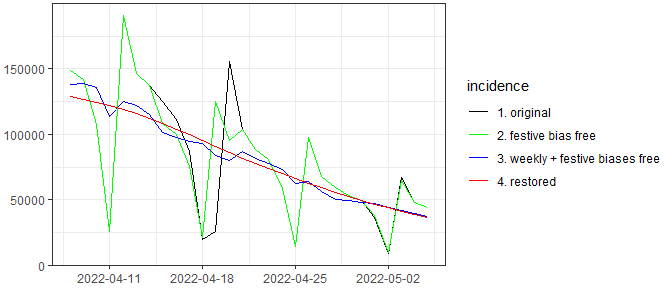Next we execute EpiInvertForecast. Notice that EpiInvertForecast has 3 parameters: (1) the outcome of the EpiInvert execution, (2) the restored incidence database and (3) the forecast option that can be “mean” or “median”.

forecast <-  EpiInvertForecast(res,restored_incidence_database,"mean")

We plot the forecast results.

 EpiInvertForecast_plot(res,forecast)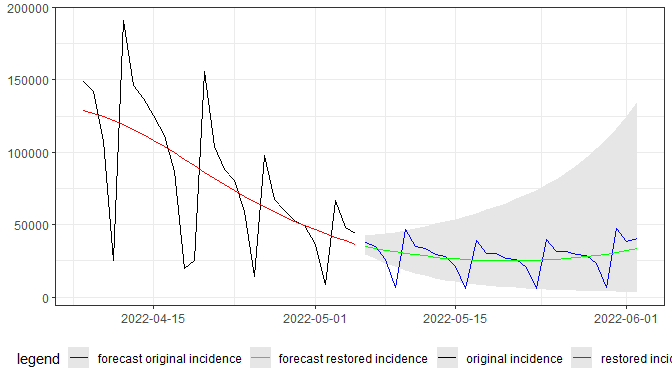Next, we use the “median” forecast option

forecast <-  EpiInvertForecast(res,restored_incidence_database,"median")
 EpiInvertForecast_plot(res,forecast)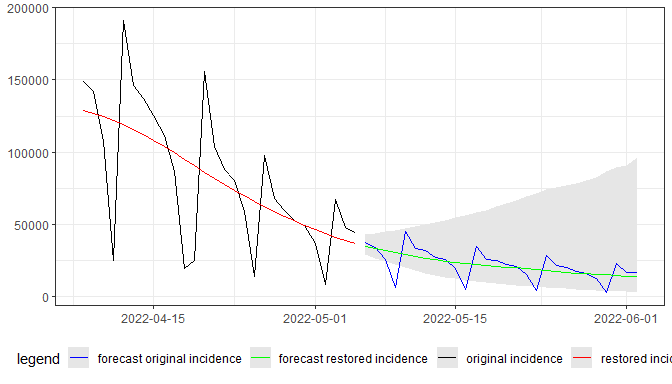We note that the predictions using the mean and median options can be quite different due to the asymmetry of the distribution of the expected value each forecast day. This asymmetry is observed in the confidence interval shown in the shaded area in the figures.

EpiInvertForecast returns a list with the following elements:

• i_restored_forecast : the forecast estimate of the restored incidence curve (the green line in the figure).

• i_restored_forecast_CI025 : the 2.5% th-percentile of the distribution of the expected error between the incidence prediction and the true value computed using the database (it corresponds to the lower limit of the shaded area in the figure).

• i_restored_forecast_CI975 : the 97.5% th-percentile of the distribution of the expected error between the incidence prediction and the true value computed using the database (it corresponds to the upper limit of the shaded area in the figure).

• dates : the date of each forecast value.

• i_original_forecast : the forecast of the original incidence curve obtained by dividing the forecast of the restored incidence curve by the weekly bias correction multiplicative factors obtained by EpiInvert (the blue line in the figure).

### Example 2

Next we apply the same procedure to the Germany data:

EpiInvert execution:

res <- EpiInvert(incidence$DEU,"2022-05-05",festives$DEU)

Plotting the results:

 EpiInvert_plot(res,"incid","2022-04-08","2022-05-05")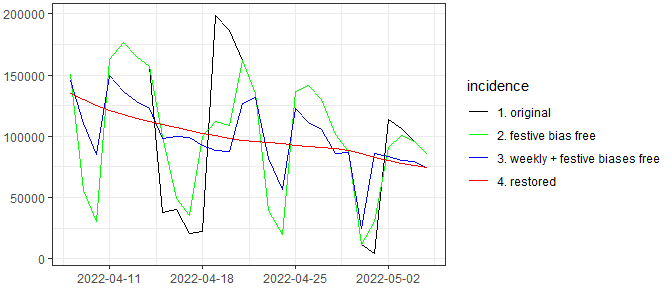EpiInvertForecast execution with the “mean” option

forecast <-  EpiInvertForecast(res,restored_incidence_database,"mean")
 EpiInvertForecast_plot(res,forecast)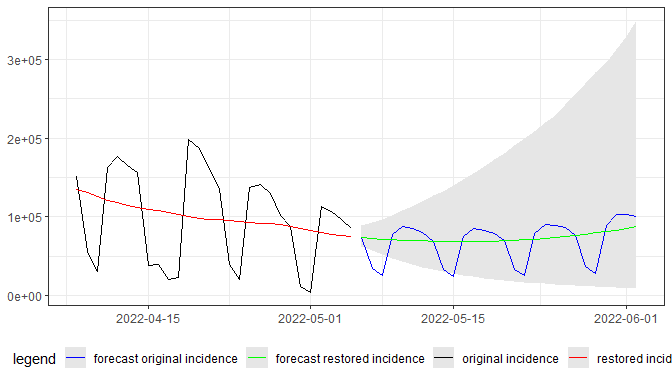EpiInvertForecast execution with the “median” option

forecast <-  EpiInvertForecast(res,restored_incidence_database,"median")
 EpiInvertForecast_plot(res,forecast)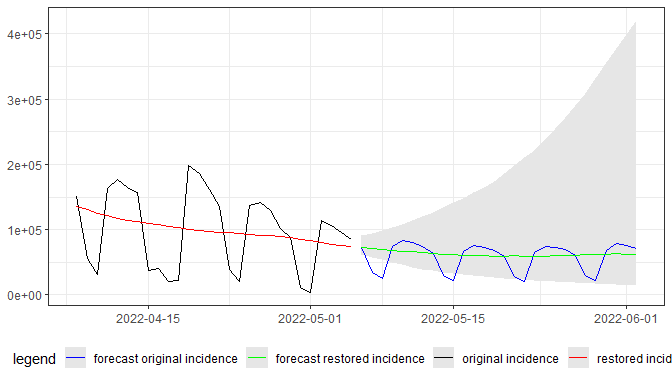### Example 3

Next we apply the same procedure to the USA data:

EpiInvert execution:

res <- EpiInvert(incidence$USA,"2022-05-05",festives$USA)

Plotting the results:

 EpiInvert_plot(res,"incid","2022-04-08","2022-05-05")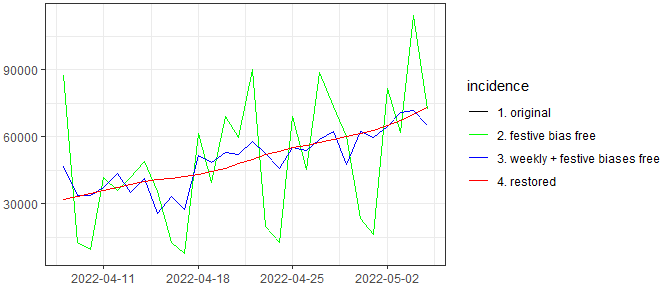EpiInvertForecast execution with the “mean” option

forecast <-  EpiInvertForecast(res,restored_incidence_database,"mean")
 EpiInvertForecast_plot(res,forecast)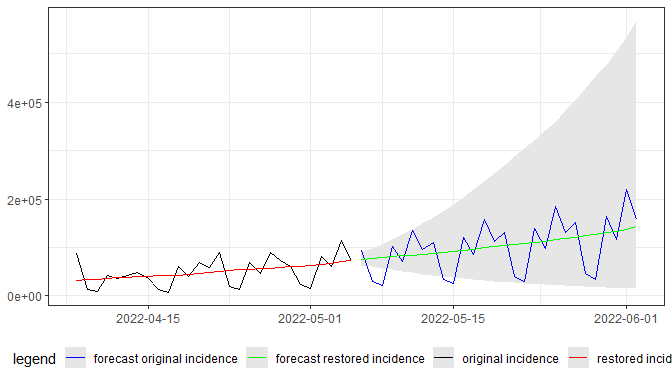EpiInvertForecast execution with the “median” option

forecast <-  EpiInvertForecast(res,restored_incidence_database,"median")
 EpiInvertForecast_plot(res,forecast)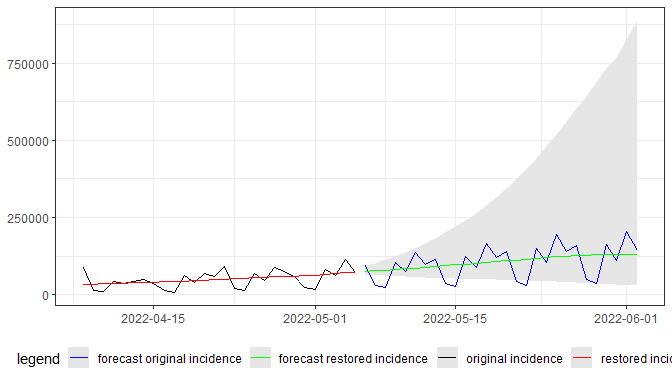### Example 4

Next we apply the same procedure to the UK data:

EpiInvert execution:

res <- EpiInvert(incidence$UK,"2022-05-05",festives$UK)

Plotting the results:

 EpiInvert_plot(res,"incid","2022-04-08","2022-05-05")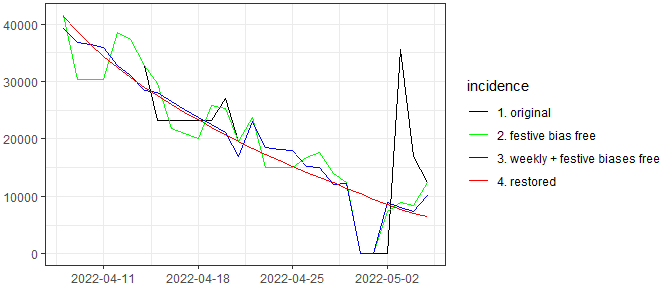EpiInvertForecast execution with the “mean” option

forecast <-  EpiInvertForecast(res,restored_incidence_database,"mean")
 EpiInvertForecast_plot(res,forecast)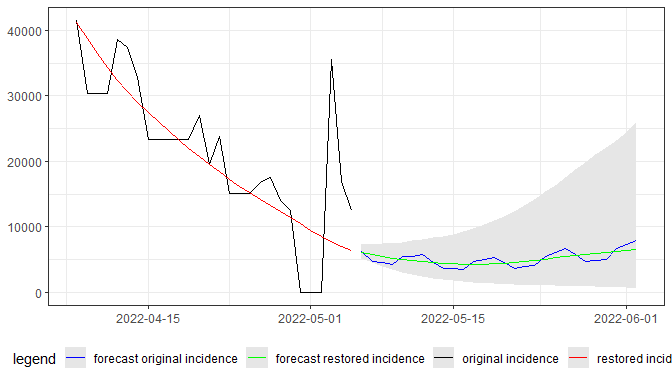EpiInvertForecast execution with the “median” option

forecast <-  EpiInvertForecast(res,restored_incidence_database,"median")
 EpiInvertForecast_plot(res,forecast)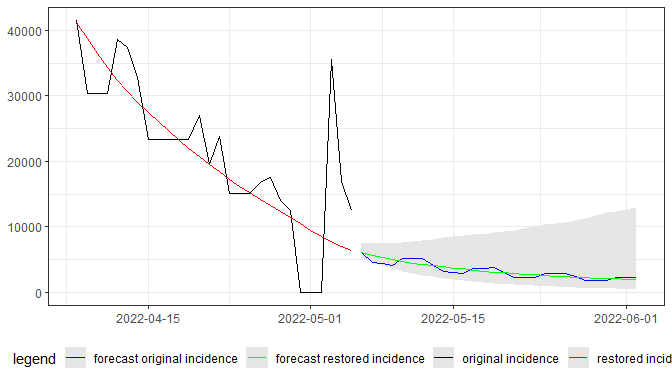## Comparing the restored incidence curve provided by EpiInvert with the 7 or 14 days average of the original daily incidence curve.

EpiInvert provides an alternative way to remove the weekly administrative bias and compute a restored incidence curve using a variational formulation of the problem. In this section, we compare the restored incidence obtained by EpiInvert with the result of the 7 or 14 days backward average of the original incidence curve, which we denote by “7-day average” or “14-day average”. We study the regularity of the estimates and the similarity between them after calculating an optimal shift between them. Festive day information is not used in this study when running EpiInvert.

We define the optimal shift, s(i,i’), between 2 incidence curves i and i’ , by minimizing the average error d(i,i’:s) :

$(1) \ \ \ \ s(i,i')=\underset{s\in\lbrack-25,25]}{\arg\min} \ \ d(i,i'\!:\!s)\equiv \frac{1}{2(T-60)}\sum_{t=30}^{T-30}\frac{|i(t)-i'(t+s)|}{(i(t)+i'(t+s))/2}+\frac{|i(t-s)-i'(t)|}{(i(t-s)+i'(t))/2}$

The average error d(i,i’:s) is computed in terms of the ratio between the difference of the incidences and the incidence magnitude.The value 30 is introduced in the formula to avoid boundary effects in the comparison. The lower the value of d(i,i’:s) the better the match between i and i’ after a shift given by s. Notice that the optimal shift is anti-symmetric, that is s(i,i’)=-s(i,i’) and d(R,R’:s) is symmetric, that is d(R,R’:s)=d(R’,R:s). To evaluate i(t-s) or i’(t+s) we use linear interpolation.

In the following figures, we show, for the different countries, the plot of the incidence curves obtained by EpiInvert, and the 7 or 14 day average applying the optimal shift. For each country, a plot is shown with the entire time period studied and another plot from March 1, 2022.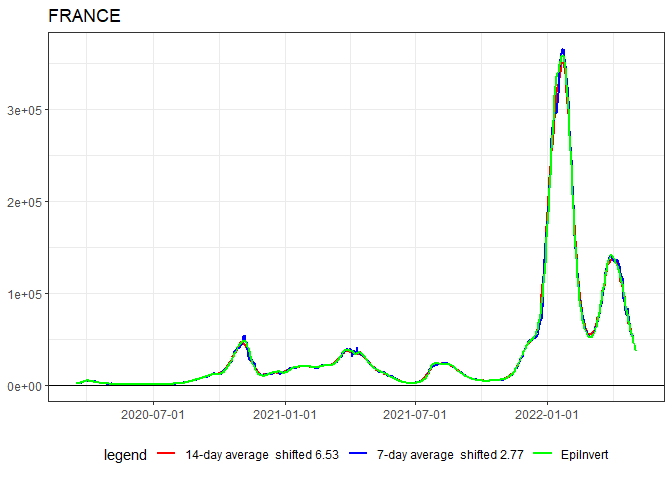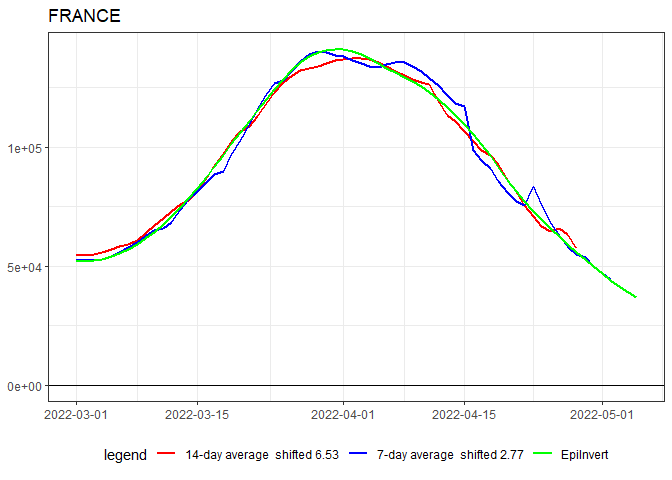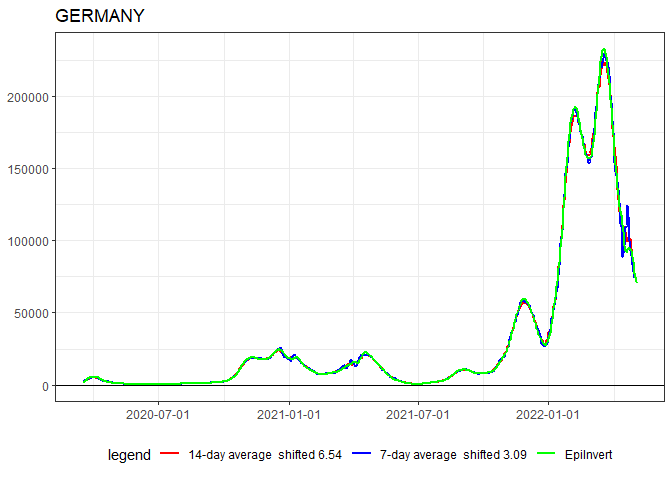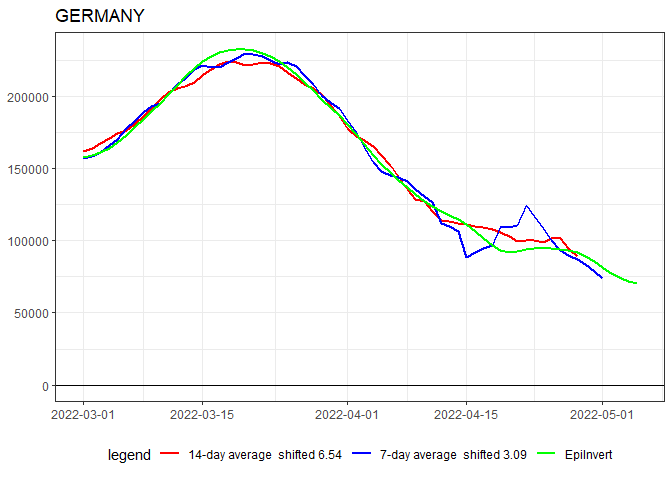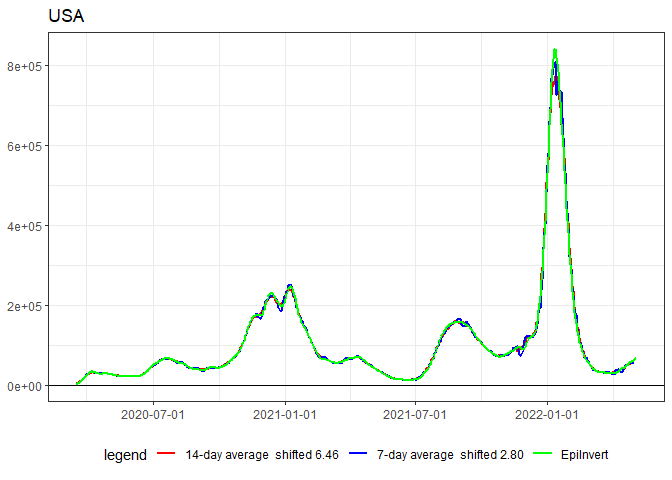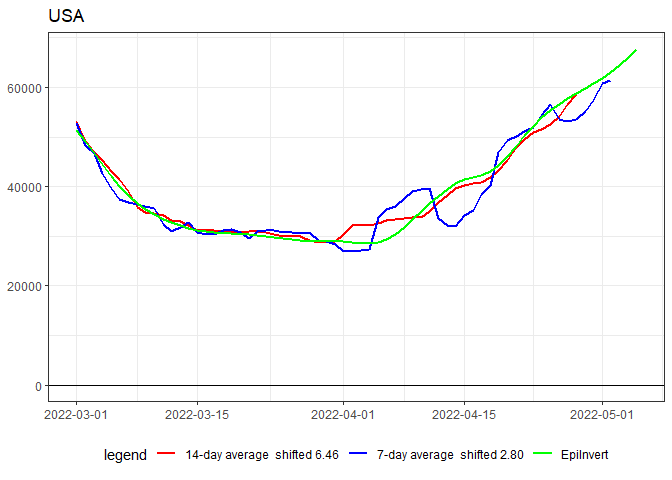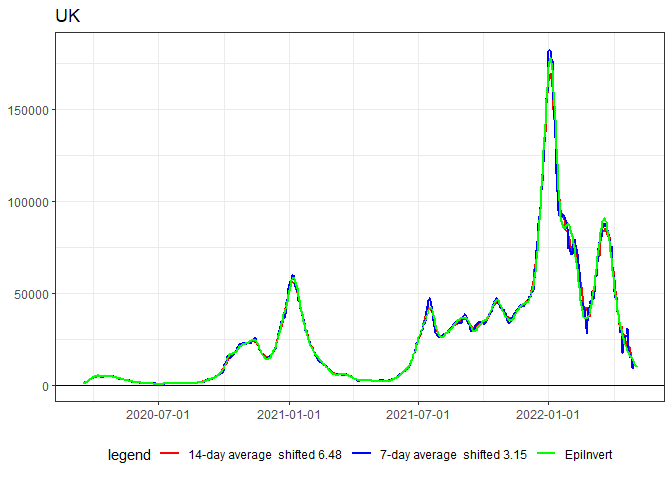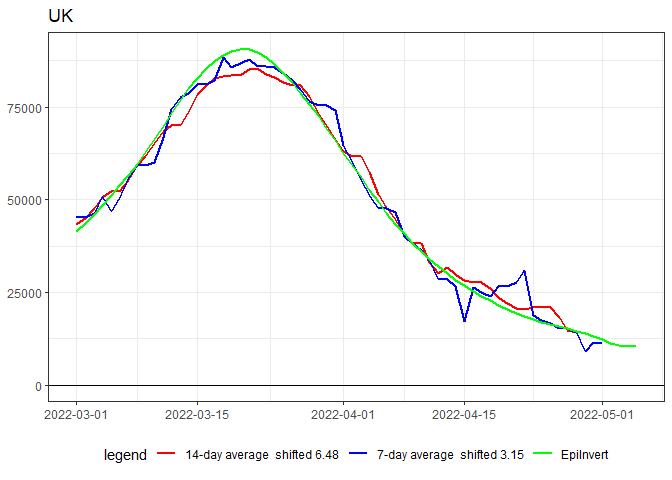We observe in these figures a very good agreement between EpiInvert and the shifted 7 and 14 days average. In the following plot we summarize the obtained results. We observe that the optimal shift is quite stable for the different countries: as expected, the shift between the 7-day average and the 14-day average is around 3.5 days. the shift between EpiInvert and the 7-day average is around 3 days and the shift between EpiInvert and the 14-day average is around 6.5 days. Concerning the average distance d(i,i’:s(i,i’)) is small in all cases. The lowest error is found when comparing EpiInvert and the 14-day average.

##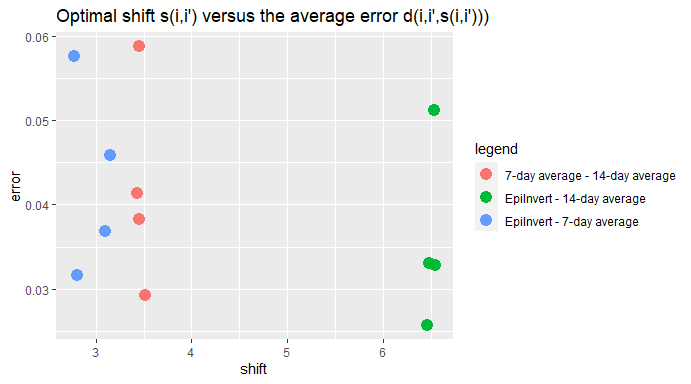To measure the regularity of the different incidence trend estimates, we use the following averages of the absolute values of the ratio of the first or second derivatives and the incidence value.

$(2) \ \ \ \ \text{FIRST DERIVATIVE AVERAGE:} \ \ ||di/dt||=\sum_{t=30}^{T-30}\frac{|i(t+h)-i(t-h)|}{2(T-60)i(t)}$

$(3) \ \ \ \ \text{SECOND DERIVATIVE AVERAGE:} \ \ ||d^2i/dt^2||=\sum_{t=30}^{T-30}\frac{|i(t+h)-2i(t)+i(t-h)|}{(T-60)i(t)}$

In the following plot we compare the regularity of the different incidence estimates. As expected, the more irregular estimation is the 7-day average. Notice that an anomalous incidence value causes two singularities in the window average, the first on the day the anomaly appears and the second on the day the window average anomaly disappears. Also, the smaller the window, the greater the effect of the anomaly. EpiInvert that uses a variational method, manages anomalies better and generates a smoother and less oscillating incidence curve, this is clearly seen in the figure where it is observed that, by far, EpiInvert has the lowest average second derivative.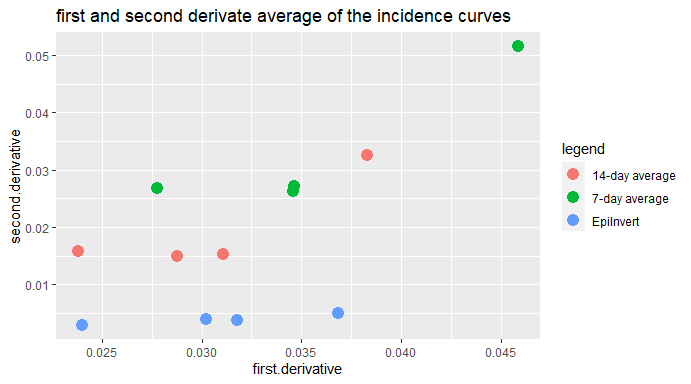The main conclusions of this comparative study are :

• EpiInvert provides an incidence trend curve similar to those provided by the usual 7 or 14 day average methods, but more up-to-date.

• EpiInvert is more similar to the 14-days average than to the 7-days average but 6.5 days closer to the present.

• EpiInvert is smoother and has much less oscillation than the usual 7 or 14 day average methods.